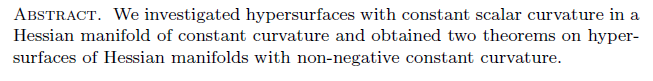Research Article

### Riemannian Hypersurfaces with Constant Scalar Curvature In a Hessian Manifolds of Constant Curvature

Year 2009, Volume 2, Issue 2, 63 - 70, 30.10.2009

### Abstract### References

•  Shima, H., Hessian manifolds of constant Hessian sectional curvature, J. Math. Soc. Japan, Vol 47, No.4, (1995), 735-753.
•  Shima, H., Homogeneous Hessian manifolds, Ann. Inst. Fourier (Grenoble),30-3, (1980), 91-128.
•  Shima, H., Vanishing theorems for compact Hessian manifolds, Ann. Inst. Fourier (Greno- ble),36-3, (1986), 183-205.
•  Shima, H., Yagi, K., Geometry of Hessian manifolds, Diff. Geo. and Its Appl. 7 (1997), 277-290.
•  Bektas, M, Yildirim, M., Kulahci, M., On hypersurfaces of Hessian manifolds with constant Hessian sectional curvature, Journal of Math. Statistics, 1 (2) ,(2005), 115-118.
•  Bektas, M., Yildirim, M., Integral inequalities for submanifolds of Hessian Manifolds with constant Hessian sectional curvature, Iranian Journal of Sci. and Tech. Trans. A , Vol.30, No.A2, (2006), 235-239.
•  Yildirim Yilmaz, M. Bektas, M., A survey on curvatures of Hessian manifolds, Chaos, Solitons and Fractals, vol.38, 3, (2008),620-630.
•  Simons, J., Minimal variety in Riemannian manifolds, Ann. of Math. 88,(1968), 62-105.
•  Chern, S. S., Do Cormo M., Kobayashi, S., Minimal submanifolds of a sphere with second fundamental form of constant length, Funct. Analysis and Related fields, Springer-Verlag, (1970),59-75.
•  Nomizu, K., Smyth, B., A formula of Simons type and hypersurfaces with constant mean curvature, J. Diff. Geo. 3,(1969), 367-377.
•  Nakagawa, H., Yokote, I., On hypersurfaces with constant scalar curvature in a Riemannian manifold of constant curvature, Kodai Math. Sem. Rep., 24, (1972), 471-481.
•  Omachi, E., Hypersurfaces with harmonic curvature in a space of constant curvature, Kodai Math. J. 9, (1986), 170-174.
Year 2009, Volume 2, Issue 2, 63 - 70, 30.10.2009

### References

•  Shima, H., Hessian manifolds of constant Hessian sectional curvature, J. Math. Soc. Japan, Vol 47, No.4, (1995), 735-753.
•  Shima, H., Homogeneous Hessian manifolds, Ann. Inst. Fourier (Grenoble),30-3, (1980), 91-128.
•  Shima, H., Vanishing theorems for compact Hessian manifolds, Ann. Inst. Fourier (Greno- ble),36-3, (1986), 183-205.
•  Shima, H., Yagi, K., Geometry of Hessian manifolds, Diff. Geo. and Its Appl. 7 (1997), 277-290.
•  Bektas, M, Yildirim, M., Kulahci, M., On hypersurfaces of Hessian manifolds with constant Hessian sectional curvature, Journal of Math. Statistics, 1 (2) ,(2005), 115-118.
•  Bektas, M., Yildirim, M., Integral inequalities for submanifolds of Hessian Manifolds with constant Hessian sectional curvature, Iranian Journal of Sci. and Tech. Trans. A , Vol.30, No.A2, (2006), 235-239.
•  Yildirim Yilmaz, M. Bektas, M., A survey on curvatures of Hessian manifolds, Chaos, Solitons and Fractals, vol.38, 3, (2008),620-630.
•  Simons, J., Minimal variety in Riemannian manifolds, Ann. of Math. 88,(1968), 62-105.
•  Chern, S. S., Do Cormo M., Kobayashi, S., Minimal submanifolds of a sphere with second fundamental form of constant length, Funct. Analysis and Related fields, Springer-Verlag, (1970),59-75.
•  Nomizu, K., Smyth, B., A formula of Simons type and hypersurfaces with constant mean curvature, J. Diff. Geo. 3,(1969), 367-377.
•  Nakagawa, H., Yokote, I., On hypersurfaces with constant scalar curvature in a Riemannian manifold of constant curvature, Kodai Math. Sem. Rep., 24, (1972), 471-481.
•  Omachi, E., Hypersurfaces with harmonic curvature in a space of constant curvature, Kodai Math. J. 9, (1986), 170-174.

### Details

Primary Language English Research Article Mahmut ERGÜT This is me October 30, 2009 Year 2009, Volume 2, Issue 2

### Cite

 Bibtex @research article { iejg599006, journal = {International Electronic Journal of Geometry}, eissn = {1307-5624}, address = {}, publisher = {Kazım İLARSLAN}, year = {2009}, volume = {2}, number = {2}, pages = {63 - 70}, title = {Riemannian Hypersurfaces with Constant Scalar Curvature In a Hessian Manifolds of Constant Curvature}, key = {cite}, author = {Y. Yılmaz, Münevver and Bektaş, Mehmet and Ergüt, Mahmut} } APA Y. Yılmaz, M. , Bektaş, M. & Ergüt, M. (2009). Riemannian Hypersurfaces with Constant Scalar Curvature In a Hessian Manifolds of Constant Curvature . International Electronic Journal of Geometry , 2 (2) , 63-70 . Retrieved from https://dergipark.org.tr/en/pub/iejg/issue/47444/599006 MLA Y. Yılmaz, M. , Bektaş, M. , Ergüt, M. "Riemannian Hypersurfaces with Constant Scalar Curvature In a Hessian Manifolds of Constant Curvature" . International Electronic Journal of Geometry 2 (2009 ): 63-70 Chicago Y. Yılmaz, M. , Bektaş, M. , Ergüt, M. "Riemannian Hypersurfaces with Constant Scalar Curvature In a Hessian Manifolds of Constant Curvature". International Electronic Journal of Geometry 2 (2009 ): 63-70 RIS TY - JOUR T1 - Riemannian Hypersurfaces with Constant Scalar Curvature In a Hessian Manifolds of Constant Curvature AU - MünevverY. Yılmaz, MehmetBektaş, MahmutErgüt Y1 - 2009 PY - 2009 N1 - DO - T2 - International Electronic Journal of Geometry JF - Journal JO - JOR SP - 63 EP - 70 VL - 2 IS - 2 SN - -1307-5624 M3 - UR - Y2 - 2022 ER - EndNote %0 International Electronic Journal of Geometry Riemannian Hypersurfaces with Constant Scalar Curvature In a Hessian Manifolds of Constant Curvature %A Münevver Y. Yılmaz , Mehmet Bektaş , Mahmut Ergüt %T Riemannian Hypersurfaces with Constant Scalar Curvature In a Hessian Manifolds of Constant Curvature %D 2009 %J International Electronic Journal of Geometry %P -1307-5624 %V 2 %N 2 %R %U ISNAD Y. Yılmaz, Münevver , Bektaş, Mehmet , Ergüt, Mahmut . "Riemannian Hypersurfaces with Constant Scalar Curvature In a Hessian Manifolds of Constant Curvature". International Electronic Journal of Geometry 2 / 2 (October 2009): 63-70 . AMA Y. Yılmaz M. , Bektaş M. , Ergüt M. Riemannian Hypersurfaces with Constant Scalar Curvature In a Hessian Manifolds of Constant Curvature. Int. Electron. J. Geom.. 2009; 2(2): 63-70. Vancouver Y. Yılmaz M. , Bektaş M. , Ergüt M. Riemannian Hypersurfaces with Constant Scalar Curvature In a Hessian Manifolds of Constant Curvature. International Electronic Journal of Geometry. 2009; 2(2): 63-70. IEEE M. Y. Yılmaz , M. Bektaş and M. Ergüt , "Riemannian Hypersurfaces with Constant Scalar Curvature In a Hessian Manifolds of Constant Curvature", International Electronic Journal of Geometry, vol. 2, no. 2, pp. 63-70, Oct. 2009